# Martin Orr's Blog

## Tate modules

Posted by Martin Orr on Sunday, 21 November 2010 at 17:32

I said after my last post that I would write something about-adic representations coming from abelian varieties. I have finally got around to doing so: here I will tell the story of how these representations are defined, and show that the Tate module is canonically isomorphic to. Next time I will relate this to Mumford-Tate groups.

### Torsion points

Let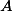be an abelian variety over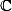of dimension. We shall look at the-torsion points of, written. The groupis isomorphic to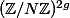.

(This fact holds over any algebraically closed field, as long asis not divisible by the characteristic, but I shall give a proof valid only overbecause this proof will show the relationship with singular homology.)

For one proof of this, recall thatis isomorphic tovia the exponential map, for some lattice. This isomorphism takesto.

Now a fundamental domain foris a parallelotope in, and the points ofinside this form an(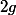times) grid. For example here is a picture of the 3-torsion points of an elliptic curve:At this point we have proved that, but in fact we have proved more: there is a canonical isomorphism betweenand. This is becauseis canonically isomorphic to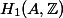and multiplication bygives an isomorphism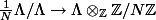.

### Tate modules

Now fix a rational prime. We want to look at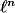-torsion points for allat once.

One way of doing this would be to take the union of all the. This gives an abelian group, but there is no interesting module structure.

Instead consider the sequence of groupswith the multiplication-by-morphisms (from the group law on the abelian variety):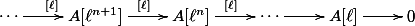Under the isomorphisms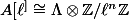, the multiplication-by-morphismbecomes the reduction-mod-map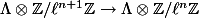.

So when we take the inverse limit, we get something isomorphic to. This is called the-adic Tate module, written.

### Galois action

Now suppose thatis defined over a number field. In this case the Tate modules carry extra structure, namely an action of.

When I talk about the "coordinates" of a point in, I shall mean the homogeneous coordinates under some embedding in projective space. These of course are completely different from the coordinates of a corresponding point in.

Since the group law onis defined by polynomials with coefficients in, there exists a set of polynomials with coefficients inwhose complex solutions are precisely the-torsion points. (Writing down these polynomials is pretty horrible but we only care that they exist.)

It follows that if we letact on the coordinates of points in, it takes-torsion points to-torsion points. Since there are only finitely many-torsion points, their coordinates must be algebraic numbers.

So the groupacts on. Furthermore, the action is compatible with the multiplication-by-morphisms, so we can pass to the limit and get an action ofon.

### Image of the Galois representation

We can interpret the image action ofonas a group homomorphismBoth sides of this homomorphism are profinite groups, so carry a natural topology. For any, the preimage underof those matrices which reduce tomodulois the set of elements of the Galois group which act trivially on. Sinceis a finite extension of, this subgroup is open in.

It follows thatis continuous, and so its imageis a compact subgroup of. This image is interesting because it tells us about the Galois groups of the fields generated by torsion points: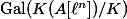is isomorphic to the reduction modof. I shall write more aboutand its conjectural relation to the Mumford-Tate group next time.

1. Images of Galois representations From Martin's Blog

In this post, I will continue to talk about the -adic representations attached to abelian varieties, and in particular the images of these representations. I will define algebraic groups approximating , which are often more convenient to work with...

2. The Hodge filtration and universal vector extensions From Martin's Blog

We will begin this post by looking at the isomorphism between the Hodge filtration H^{0,-1}(A) \subset H_1(A, \mathbb{C}) of a complex abelian variety A and the natural filtration ... on the tangent space to the universal vector extension of A. ...

1. Barinder said on Sunday, 21 November 2010 at 21:35 :

Nice Post. I've been thinking about this recently; in particular, I wanted to say that for only finitely many primesis the image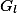contained in a Borel subgroup of. Is this true in general? What about for the mod-representations that one can get from the-adic one? In my case, I had a bunch of-adic representations coming from weight 2 newforms (of any level), and wanted to say something like, almost always, the image is inside a Borel subgroup.

I'm looking forward to your next post.

2. Martin Orr said on Monday, 22 November 2010 at 10:58 :

Are your abelian varieties defined over number fields (as opposed to say local fields)? From what I remember about the varieties associated to modular forms, the answer is yes.

Then I think that, as long as your variety is not CM, the image is never contained in a Borel subgroup. A quick sketch: let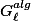be the Zariski closure of the image ofin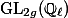. By Faltings the connected component of this is reductive. If it is contained in a Borel then it is solvable. A group which is both reductive and solvable must be commutative. If the connected component ofis commutative, then the abelian variety is CM.

In the CM case it matters what you take as your base field. If the base field is large enough (something like if it contains the Galois closure of the CM field) thenis commutative, so is contained in a Borel.